Courses

# Cauchy Residue Theorem - Mathematical Methods of Physics, UGC - NET Physics Physics Notes | EduRev

## Physics for IIT JAM, UGC - NET, CSIR NET

Created by: Akhilesh Thakur

## Physics : Cauchy Residue Theorem - Mathematical Methods of Physics, UGC - NET Physics Physics Notes | EduRev

The document Cauchy Residue Theorem - Mathematical Methods of Physics, UGC - NET Physics Physics Notes | EduRev is a part of the Physics Course Physics for IIT JAM, UGC - NET, CSIR NET.
All you need of Physics at this link: Physics

Complex Numbers and Complex Functions

A complex number z can be written as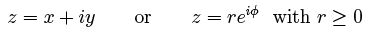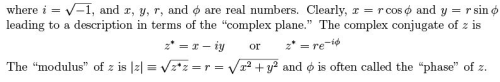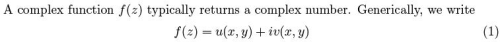for purposes of proofs or illustrations. The behavior of the (real) functions u(x, y) and v(x, y) are critical for classifying complex functions, as seen when we consider taking derivatives.

Dierentiation and Analyticity

We de ne the derivative f'(z) = df =dz of a complex function f (z) in the same was as we do for the derivatives of real functions. That is, for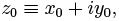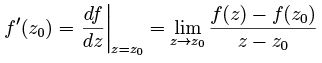However, there is clearly an ambiguity, depending on whether we approach z0 along the line y = y0 or along x = x0. (Of course, we could also say the ambiguity is along any line of constant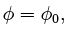but it is sucient to consider just two orthogonal directions.) That is,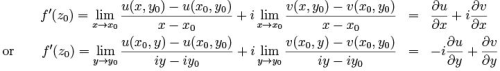Therefore, in order to remove the ambiguity and have a consistent de nition of the derivative,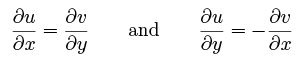(2)

These are called the Cauchy-Riemann conditions. A function f (z) which satis es these rather restrictive conditions is called analytic. Indeed, analytic functions have very many applications in physics, and we will merely scratch the surface here.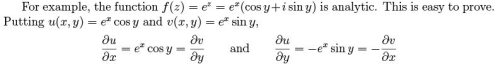so the Cauchy-Riemann conditions (2) are satised.

It is simple to show that f (z ) = az is analytic, where a is a complex constant. It is also not hard to show that the product of two analytic functions is analytic, so any function of the form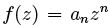where n is a non-negative integer, is also analytic. Of course, any sum of analytic functions is analytic, so we see that any polynomial in z is analytic in the entire complex plane.

These examples beg the question: If a function f (z) can be written explicitly in terms of z , is it analytic? The answer is \Yes." To see this, realize that instead of x and y, we could always write a complex function in terms of z and z* using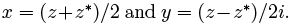Now consider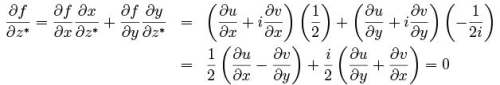so long as the Cauchy-Riemann conditions (2) are satis ed. That is, if the expression for f (z ) contains only z (and not z*) then the function is analytic.

There is some common terminology. A function f (z) need not be analytic in the entire complex plane. (If it is, we called the function \entire.") If it is analytic at a point z0 then we call that a \regular point." Otherwise, z0 is called a \singular point." Much of our discussion of complex integration will focus on the notion of singular points.

Integration and Series Expansion

Similarly to dierentiation, we approach integration of complex functions the same way as with real functions, but we need to be aware that there is now an arbitrariness of the "path" of integration. With dz = dx + idy and using using (1), we have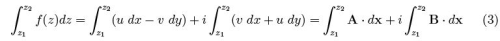where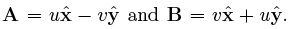So, we can now think of the two integrals on the right as real integrals of vector functions over curves in the xy plane. However, if we invoke Stokes' Theorem, these become integrals of the curls, and using (2), we nd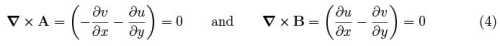and each of the two integrals on the right in (3) are path-independent. Hence, the integral of an analytic complex function f (z) is path-independent and can be unambiguously de ned.

From here on, we assume all functions to be analytic unless explicitly noted otherwise. It is obvious from (3) that, when integrating around a closed path C ,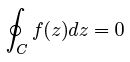which is known as the Cauchy-Goursat Theorem. We will be exploring circumstances where the integrand is explicitly singular at one or more points.

For the rst example, we prove the Cauchy Integral Formula, namely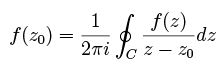(5)

where C is a closed contour in the complex plane that contains the point z0 and traversed in the counterclockwise direction. We can break up a contour C into something that looks like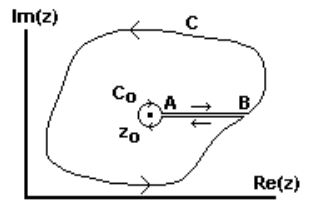where C0 is a tiny circular contour around the singular point, but in the clockwise direction.

That is, we replace C with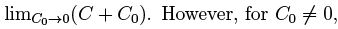the new contour C does not include the singular point, so by (4) we write (5) as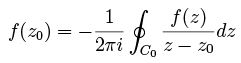(6)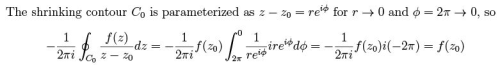proving the Cauchy Integral Formula (5). A trivial, but suggestive, rewriting of (5) gives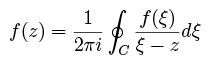(7)

which leads to a convenient way to write the derivatives of a complex function, namely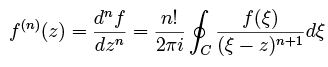(8)

Now consider the series expansion of an analytic function f (z). We would naturally write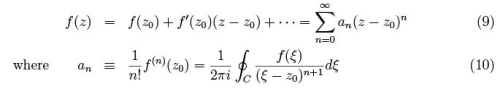Such a Taylor Series expansion works out as expected, but the curve C speci es regions in which the series converges.

This idea can be expanded to include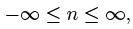still using the right side of (10) to de ne an, and with modi ed regions of convergence. Such an expansion is called a Laurent Series. It clearly is not, in general, an analytic function because of poles that appear for n < 0. These, however, lead us to one of the most important theorems of complex analysis, so far as mathematical physics is concerned.

The Cauchy Residue Theorem

Let g(z) have an isolated singularity at z = z0. If the Laurent expansion can be written as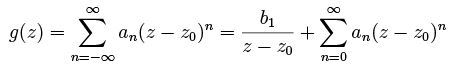(11)

then we say that g(z) has a \simple pole" at z = z0. Higher order poles are possible, but we're not going to consider them here.

Consider a contour C within the radius of convergence of g(z). Separate the integral of g(z) around this contour into two terms, one for each of the two terms on the right in (11).
The second term is a polynomial in z ; therefore it is analytic and the integral is zero. Recall that we reduced the contour to a small circle around the pole in order to prove the Cauchy Integral Formula. We can do the same thing here, and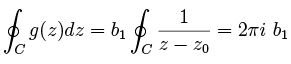(12)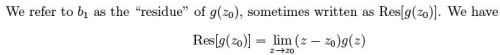for a simple pole at z0.

If there are more than one simple pole within the contour C , this result is easy to generalize. Instead of redrawing the contour with a small loop about the single pole, do it for all N poles within the contour. The result is clearly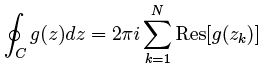(13)

We refer to this as the Cauchy Residue Theorem. It is widely used in mathematical physics.

The usefulness of the Residue Theorem can be illustrated in many ways, but here is one important example. It is a warm-up to evaluating the integral (6.2.9) in Modern Quantum Mechanics, 2nd Ed. The exercise is to evaluate the integral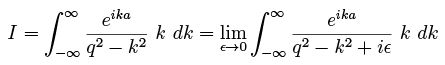(14)

where k, a, q, and ε > 0 are all real variables. We use the second version above because this moves the singularities at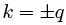off the real axis. To be sure, we could have moved off the real axis by using -iε instead of +iε, and in fact, this would give us a dierent answer. A physical rationale is needed to justify one sign or the other. Leave that for a physics course.

We can evaluate (14) using contour integration by rst allowing k to be complex and then noting that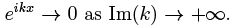Therefore (14) can be rewritten as an integral over a semicircular contour C that runs (counter clockwise) along the Re(k) axis and closes as a semicircle in the Im(k) > 0 plane. Then for ε → 0, the integrand in (14) has poles at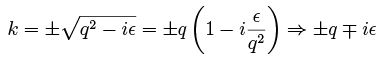where we rede ne ε  (with q > 0) so that it is still small and has the same sign.

The pole at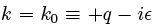does not matter to us, since it is outside the integration contour. However, the pole at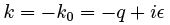is inside, so we use the Residue Theorem to write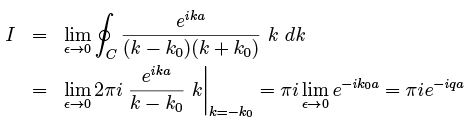159 docs

,

,

,

,

,

,

,

,

,

,

,

,

,

,

,

,

,

,

,

,

,

,

,

,

;